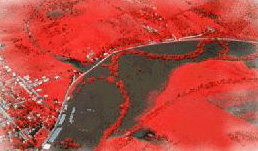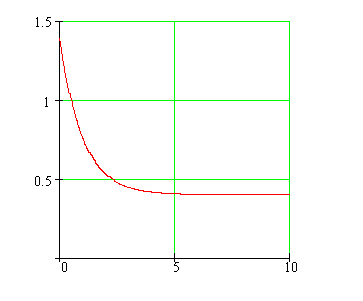Calculus on Demand at Dartmouth College Lecture 17 | Index | Lecture 19 Lecture 18## Resources

Math 3 Course Syllabus
Practice Exams

# Contents

In this lecture we continue with the discussion of separable differential equations. We also consider exponential growth and decay, important models in real-world applications.

### Quick Question

Is the town population depicted in the following graph, growing, decreasing, or staying the same over the last ten years?### Outline

Outlines for
Exponential Growth and Decay
Separable Differential Equations

### Textbook

Exponential Growth and Decay
Separable Differential Equations